# You generated liposomes with phospholipid and cholesterol from a solution containing NaCl, glucose, and glycerol. You...

You generated liposomes with phospholipid and cholesterol from a solution containing NaCl, glucose, and glycerol. You precipitated the liposomes and transferred them into a solution containing distilled water. Would this liposome generate a membrane potential?

YES OR NO?

Would this liposome generate a membrane potential?

Yes

Differences in the concentrations of ions on opposite sides of a cellular membrane result a voltage that is called the membrane potential.

#### Earn Coin

Coins can be redeemed for fabulous gifts.

Similar Homework Help Questions
• ### If you placed a dialysis bag containing NaCl solution, starch solution and glucose solution in a...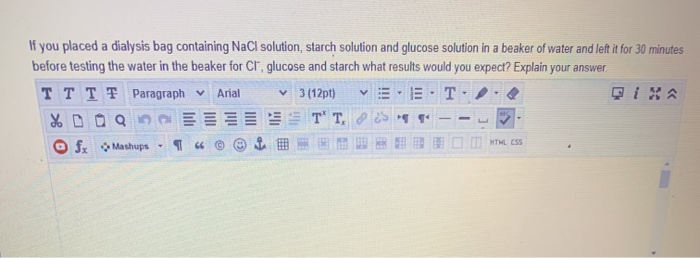If you placed a dialysis bag containing NaCl solution, starch solution and glucose solution in a beaker of water and left it for 30 minutes before testing the water in the beaker for Cr, glucose and starch what results would you expect? Explain your answer TTTF Paragraph v Arial 3(12pt) ET %DOQ 25" fx Mashups BIMMLESS T' T.

• ### The following five beakers, each containing a solution of sodium chloride (NaCl, also known as table...

The following five beakers, each containing a solution of sodium chloride (NaCl, also known as table salt), were found on a lab shelf: Beaker Contents 1 200 mL. of 1.50 M NaCl solution 2 100 mL. of 3.00 M NaCl solution 3 150 mL. of solution containing 22.5 g of NaCl 4 100 mL. of solution containing 22.5 g of NaCl 5 300 mL. of solution containing 0.450 mol NaCl Arrange the solutions in order of decreasing concentration. Rank from...

• ### At equal concentrations, would a nonelectrolyte (e.g. glucose) or electrolyte (e.g. NaCl) containing solution have lower...

At equal concentrations, would a nonelectrolyte (e.g. glucose) or electrolyte (e.g. NaCl) containing solution have lower freezing point? Why?

• ### How would you make a 350 ml solution of 2% Glucose (MW = 180.16) in water?...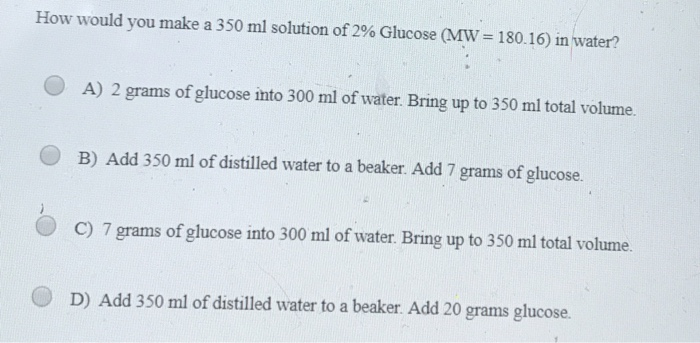How would you make a 350 ml solution of 2% Glucose (MW = 180.16) in water? A) 2 grams of glucose into 300 ml of water. Bring up to 350 ml total volume. B) Add 350 ml of distilled water to a beaker. Add 7 grams of glucose. C) 7 grams of glucose into 300 ml of water. Bring up to 350 ml total volume. D) Add 350 ml of distilled water to a beaker. Add 20 grams glucose.

• ### You are given a 2M stock solution of NaCl and are asked to make up 100...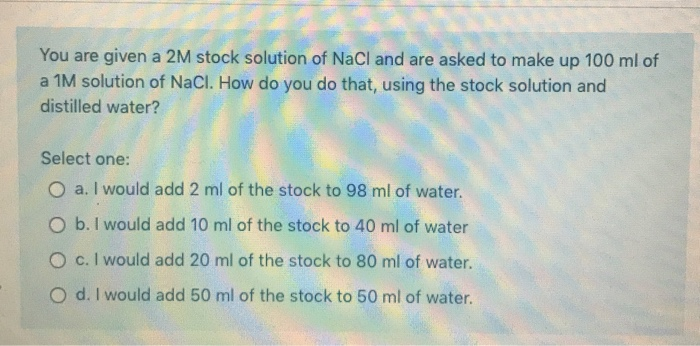You are given a 2M stock solution of NaCl and are asked to make up 100 ml of a 1M solution of NaCl. How do you do that, using the stock solution and distilled water? Select one: O a. I would add 2 ml of the stock to 98 ml of water. O b. I would add 10 ml of the stock to 40 ml of water O c. I would add 20 ml of the stock to 80 ml...

• ### Calculate the number of ATP generated from the complete oxidation of one molecule of glucose to...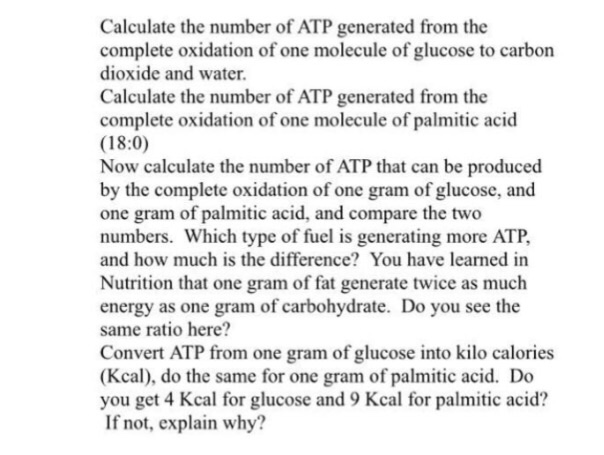Calculate the number of ATP generated from the complete oxidation of one molecule of glucose to carbon dioxide and water. Calculate the number of ATP generated from the complete oxidation of one molecule of palmitic acid (18.0) Now calculate the number of ATP that can be produced by the complete oxidation of one gram of glucose, and one gram of palmitic acid, and compare the two numbers. which type of fuel is generating more ATP. And how much is the...

• ### Calculate the number of ATP generated from the complete oxidation of one molecule of glucose to...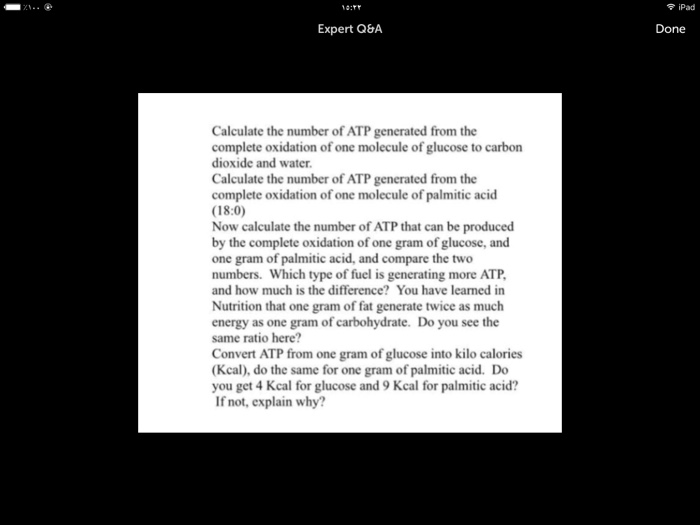Calculate the number of ATP generated from the complete oxidation of one molecule of glucose to carbon dioxide and water. Calculate the number of ATP generated from the complete oxidation of one molecule of palm tic acid Now calculate the number of ATP that can be produced by the complete oxidation of one gram of glucose, and one gram of palm tic acid, and compare the two numbers. Which type of fuel is generating more ATP. And how much is...

• ### QUESTION5 Starting with a stock solution containing 60 mg/dL of glucose, and using dH2O as your...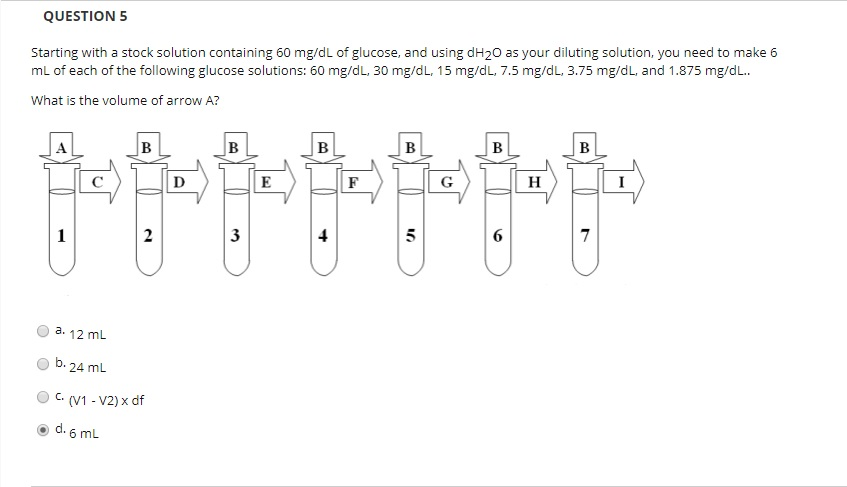QUESTION5 Starting with a stock solution containing 60 mg/dL of glucose, and using dH2O as your diluting solution, you need to make 6 mL of each of the following glucose solutions: 60 mg/dL, 30 mg/dL, 15 mg/dL, 7.5 mg/dL, 3.75 mg/dL, and 1.875 mg/dL.. What is the volume of arrow A? 3 O a 12 mL Ob. 24 mL C(V1-V2) x df ⓔd.6mL QUESTION6 Starting with a stock solution containing 60 mg/dL of glucose, and using dH20 as your diluting...

• ### Pour 2.5 mL of the 1M glucose (C6H12O6) solution from the flask into the 100 mL...

Pour 2.5 mL of the 1M glucose (C6H12O6) solution from the flask into the 100 mL volumetric flask. Use the “dilution equation” to determine the volume in mL for the required 0.025M solution, given that the original solution is 1M and you used 2.5 mL Fill the volumetric flask to the 100 mL mark with distilled water. Note: The flask already contains a volume of 2.5 mL. 1. Compute the volume of the diluted 0.025 M solution.   2. What volume...

• ### Membrane Separation II Reverse Osmosis (a) Derive van't Hoff's equation (osmotic pressure π-CRT). (b) A feed solution containing 5g NaCl/L (ρ-1001kg/m3) is purified using a reverse osmosis sy...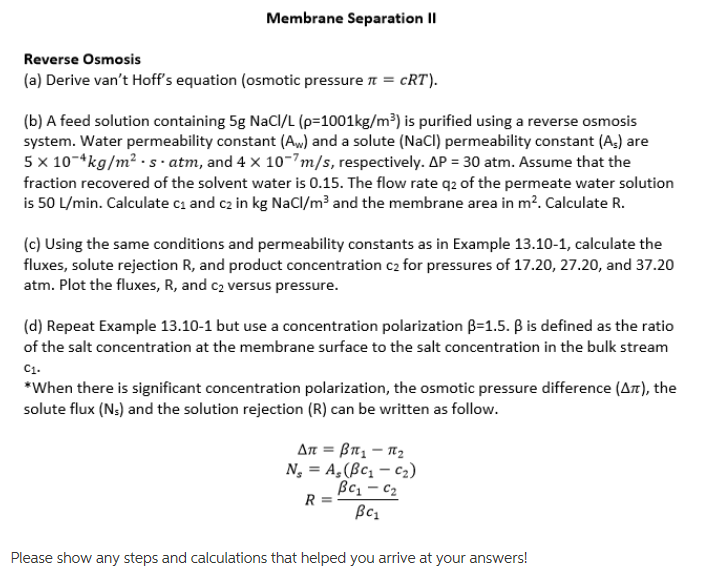Membrane Separation II Reverse Osmosis (a) Derive van't Hoff's equation (osmotic pressure π-CRT). (b) A feed solution containing 5g NaCl/L (ρ-1001kg/m3) is purified using a reverse osmosis system. Water permeability constant (Aw) and a solute (NaCI) permeability constant (As) are 5 x 10 kg/m2s atm, and 4 x 10-7m/s, respectively. AP 30 atm. Assume that the fraction recovered of the solvent water is 0.15. The flow rate q2 of the permeate water solution is 50 L/min. Calculate ci and c2...